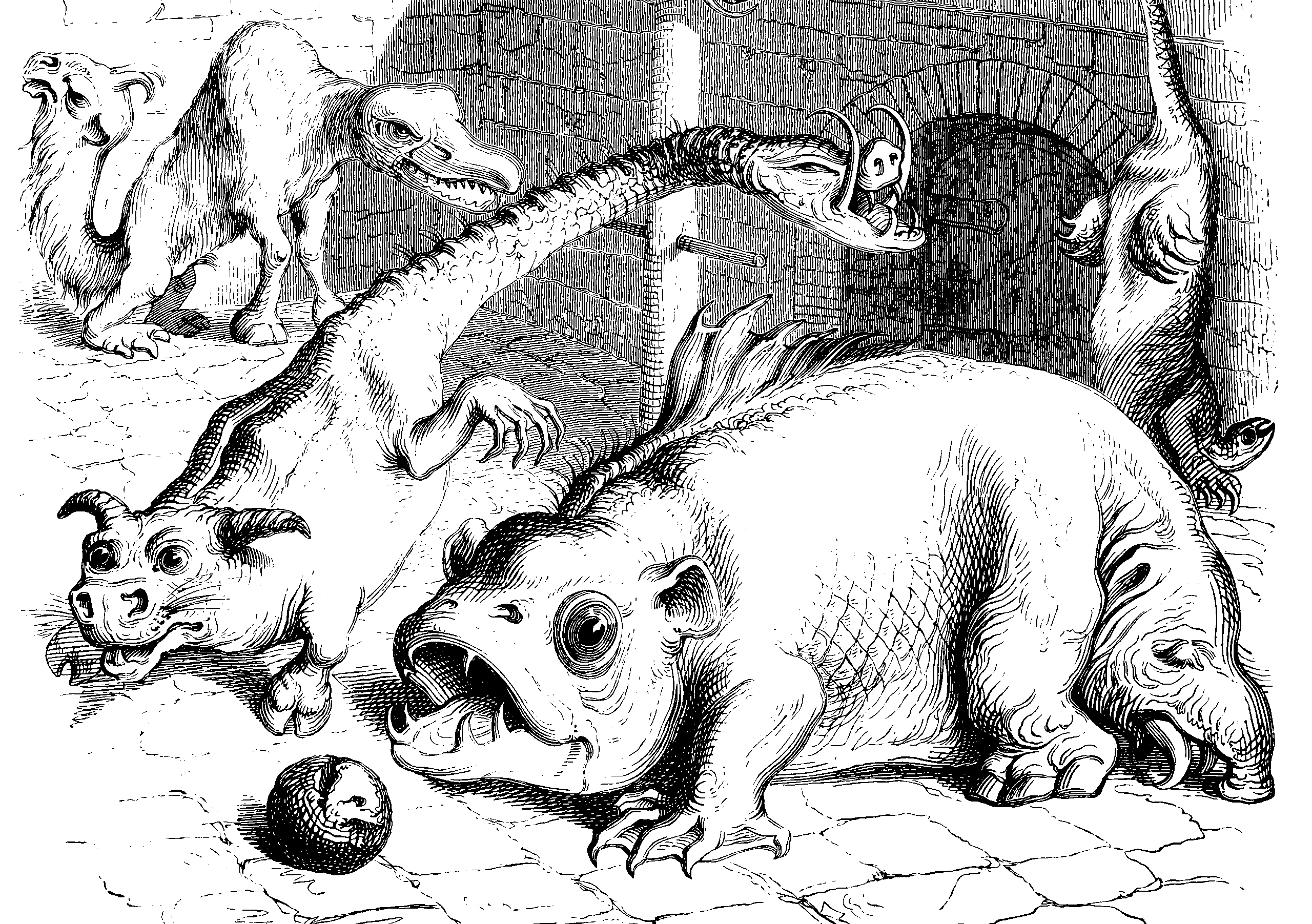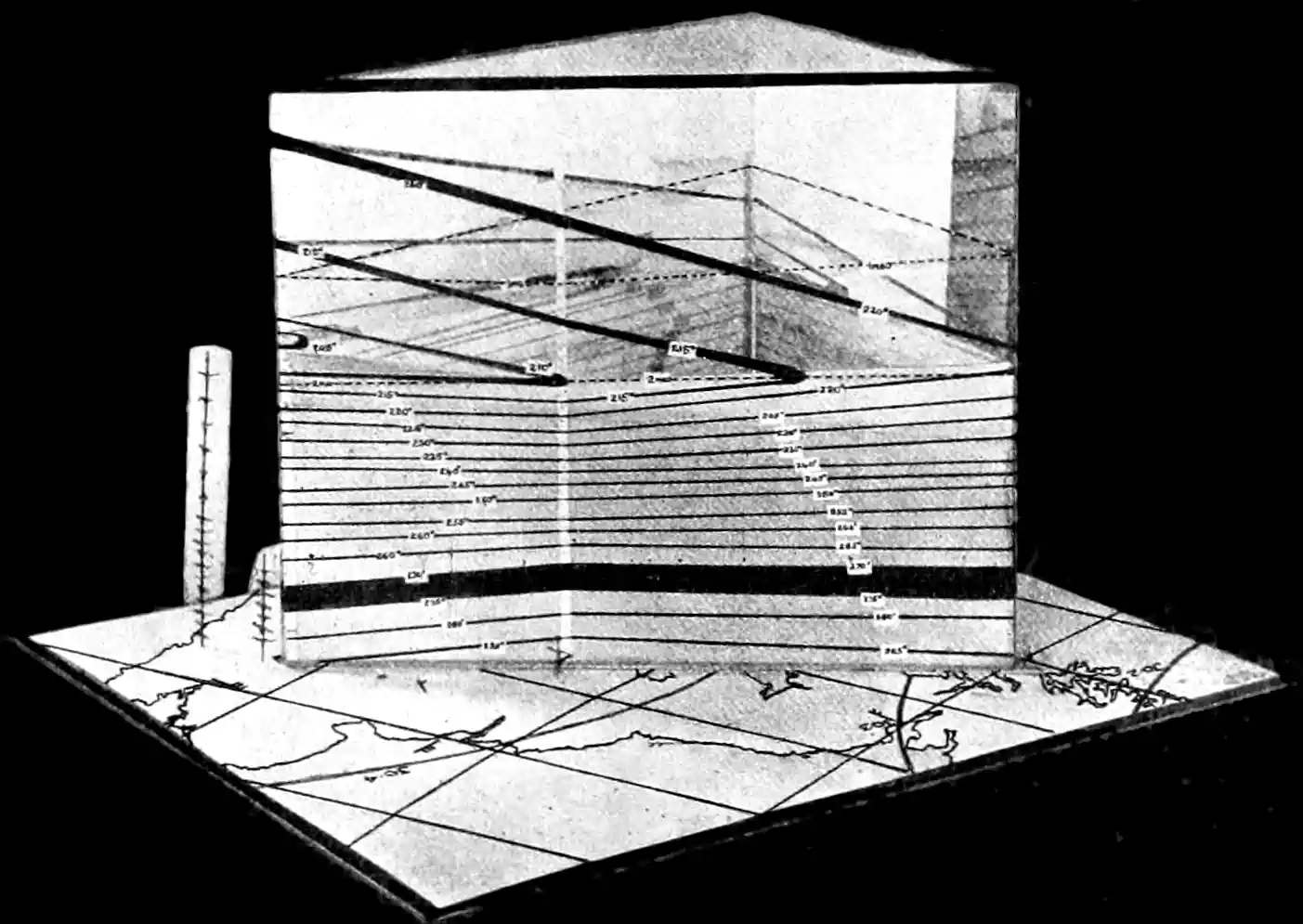# Bayesian inverse problems in function space

## a.k.a. Bayesian calibration, model uncertainty for PDEs and other wibbly, blobby thingsInverse problems where the unknown parameter is in some function space. For me this usually implies a spatiotemporal model, usually in the context of PDE solvers, particularly approximate ones.

Suppose I have a PDE, possibly with some unknown parameters in the driving equation. I can do an adequate job of predicting the future behaviour of that system if I somehow know the governing equations, their parameters, and the current state. But what if I am missing some information? What if I wish to simultaneously infer some unknown inputs? Let us say, the starting state? This is the kind of problem that we refer to as an inverse problem. Inverse problems arise naturally in tomography, compressed sensing, deconvolution, inverting PDEs and many other areas.

The thing that is special about PDEs is that they have a spatial structure, much more than structured than the low-dimensional inference problems that statisticians traditionally looked at, and so it is worth reasoning them through from first principles.

In particular I would liked to work through enough notation here that I can understand the various methods used to solve these inverse problems, for example, simulation-based inference, MCMC methods, GANs or variational inference.

Generally, I am interested in problems that use some kind of probabilistic network so that we can not just guess the solution but also do uncertainty quantification.

## Discretisation

First step is imagining how we can handle this complex problem in a finite computer. Lassas, Saksman, and Siltanen (2009) introduce a nice notation for this, which I use here. This connects the problem of inference to the problem of sampling theory, via the realisation that we need to discretize the solution in order to compute it.

I also wish to ransack their literature review:

The study of Bayesian inversion in infinite-dimensional function spaces was initiated by Franklin (1970) and continued by Mandelbaum (1984);Lehtinen, Paivarinta, and Somersalo (1989);Fitzpatrick (1991), and Luschgy (1996). The concept of discretization invariance was formulated by Markku Lehtinen in the 1990’s and has been studied by D’Ambrogi, Mäenpää, and Markkanen (1999);Sari Lasanen (2002);S. Lasanen and Roininen (2005);Piiroinen (2005). A definition of discretization invariance similar to the above was given in Lassas and Siltanen (2004). For other kinds of discretization of continuum objects in the Bayesian framework, see Battle, Cunningham, and Hanson (1997);Niinimäki, Siltanen, and Kolehmainen (2007)… For regularization based approaches for statistical inverse problems, see Bissantz, Hohage, and Munk (2004);Engl, Hofinger, and Kindermann (2005);Engl and Nashed (1981);Pikkarainen (2006). The relationship between continuous and discrete (non-statistical) inversion is studied in Hilbert spaces in Vogel (1984). See Borcea, Druskin, and Knizhnerman (2005) for specialized discretizations for inverse problems.

The insight is that there are two discretizations that are relevant, the discretization of the measurements and the discretization of the representation of a solution. We see naturally that we need to use one discretization, $$P_{k}$$ to handle the finiteness of our measurements, and another, $$T_{n}$$, to characterise the finite dimensionality of our solution.

Consider a quantity $$U$$ observed via some indirect, noisy mapping $M=A U+\mathcal{E},$ where $$A$$ is an operator and $$\mathcal{E}$$ is some mean-zero noise. We call this the continuum model. Here $$U$$ and $$M$$ are functions defined on subsets of $$\mathbb{R}^{d}$$. We start by assuming $$A$$ is linear smoothing operator - think of convolution with some kernel. We intend to use Bayesian inversion to deduce information about $$U$$ from measurement data concerning $$M$$. We write these using random function notations: $$U(x, \omega), M(y, \omega)$$ and $$\mathcal{E}(y, \omega)$$ are random functions with $$\omega \in \Omega$$ pulled some probability space $$(\Omega, \Sigma, \mathbb{P})$$. $$x$$ and $$y$$ denote the function arguments, i.e. range over the Euclidean domains. These objects are all continuous; we explore the implications of discretising them.

Next we introduce the practical measurement model, which is the first kind of discretisation. We assume that this measurement device provides us with a $$k$$-dimensional realization $M_{k}=P_{k} M=A_{k} U+\mathcal{E}_{k},$ where $$A_{k}=P_{k} A$$ and $$\mathcal{E}_{k}=P_{k} \mathcal{E}$$. $$P_{k}$$ is a linear operator describing the measurement process. Typically it will look something like $$P_{k} v=\sum_{j=1}^{k}\left\langle v, \phi_{j}\right\rangle \phi_{j}$$ for some orthogonal basis $$\{\phi_{j}\}_j$$. For simplicity we take $$P_{k}$$ to be a projection onto a $$k$$-sized orthogonal basis. Realized measurements are written $$m=M\left(\omega_{0}\right)$$, for some $$\omega_{0} \in \Omega$$. Projected meaurement vectors are similarly written $$m_{k}=M_{k}\left(\omega_{0}\right)$$.

In this notation, the inverse problem is: given a realization $$M_{k}\left(\omega_{0}\right)$$, estimate the distribution of $$U$$.

We cannot represent that distribution yet because $$U$$ is a continuum object. So we introduce another discretization, via another projection operator $$T_n$$ which maps $$U$$ to a $$n$$-dimensional space, $$U_n:=T_n U$$. This gives us the computational model, $M_{k n}=A_{k} U_{n}+\mathcal{E}_{k}.$

I said we would use this to understand this in Bayesian terms. We manufacture some prior density $$\Pi_{n}$$ over discretisations, $$U_{n}$$.

TBC

## Very nearly exact methods

For specific problems there are specific methods, for example F. Sigrist, Künsch, and Stahel (2015b) and Liu, Yeo, and Lu (2020), for advection/diffusion equations.

## Approximation of the posterior

Generic models are more tricky and we usually have to approximation _some_thing. See Bao et al. (2020);Jo et al. (2020);Lu et al. (2021);Raissi, Perdikaris, and Karniadakis (2019);Tait and Damoulas (2020);Xu and Darve (2020);Yang, Zhang, and Karniadakis (2020);D. Zhang, Guo, and Karniadakis (2020);D. Zhang et al. (2019).

## Bayesian nonparametrics

Since this kind of problem naturally invites functional parameters, we can also imagine considering it in the context of Bayesian nonparametrics, which has a slightly different notation than you usually see in Bayes textbooks. I suspect that there is a useful role for diverse Bayesian nonparametrics here, esp non-smooth random measures, but the easiest of all is Gaussian process, which I handle next.

## Gaussian process parameters

Alexanderian (2021) states a ‘well-known’ result, that the solution of a Bayesian linear inverse problem with Gaussian prior and noise models is a Gaussian posterior $$\mu_{\text {post }}^{y}=\mathcal{N}\left(m_{\text {MAP }}, \mathcal{C}_{\text {post }}\right)$$, where $\mathcal{C}_{\text {post }}=\left(\mathcal{F}^{*} \boldsymbol{\Gamma}_{\text {noise }}^{-1} \mathcal{F}+\mathcal{C}_{\text {pr }}^{-1}\right)^{-1} \quad \text { and } \quad m_{\text {MAP }}=\mathcal{C}_{\text {post }}\left(\mathcal{F}^{*} \boldsymbol{\Gamma}_{\text {noise }}^{-1} \boldsymbol{y}+\mathcal{C}_{\text {pr }}^{-1} m_{\text {MAP }}\right).$

Note the connection to Gaussian belief propagation.

## Finite Element Models and belief propagationFinite Element Models of PDEs of PDEs (and possibly other representations? Orthogonal bases generally?) can be expressed through locally-linear relationships and thus analysed using Gaussian Belief Propagation . Note that in this setting, there is nothing special about the inversion process. Inference proceeds the same either forward or inversely, as a variational message passing algorithm.

## Score-based generative models

a.k.a. neural diffusions etc. Powerful, probably a worthy default starting point for new work

### No comments yet. Why not leave one?

GitHub-flavored Markdown & a sane subset of HTML is supported.